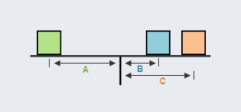# If A = 11 ft., B = 1 ft., C = 8 ft., the green box weighs 30 lbs. and the blue box weighs 50 lbs., what does the orange box have to weigh for this lever to balance?35 lbs.

Explanation

In order for this lever to balance, the torque acting on each side of the fulrum must be equal. So, the torque produced by A must equal the torque produced by B and C. Torque is weight x distance from the fulcrum which means that the following must be true for the lever to balance:
$$f_A d_A=f_B d_B+f_C d_C$$
For this problem, this equation becomes:
$$30 \mathrm{lbs} . \times 11 \mathrm{ft} .=50 \mathrm{lbs} . \times 1 \mathrm{ft} .+\mathrm{f}_{\mathrm{c}} \times 8 \mathrm{ft}$$
$$330 \mathrm{ft} . ~ I b s .=50 \mathrm{ft} . ~ I b s .+f_c \times 8 \mathrm{ft}$$

$$\mathrm{f}_{\mathrm{C}}=\frac{330 \mathrm{ft} . \mathrm{lbs} .-50 \mathrm{ft} . \mathrm{lbs} .}{8 \mathrm{ft} .}=\frac{280 \mathrm{ft} . \mathrm{lbs} .}{8 \mathrm{ft} .}=35 \mathrm{lbs}$$